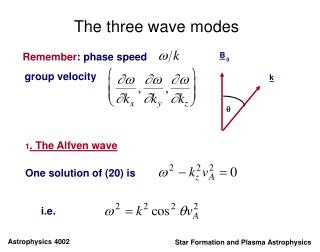DownloadDownload PresentationThe three wave modes

# The three wave modes

Télécharger la présentation## The three wave modes

- - - - - - - - - - - - - - - - - - - - - - - - - - - E N D - - - - - - - - - - - - - - - - - - - - - - - - - - -
##### Presentation Transcript

1. B 0 k q The three wave modes Remember: phase speed group velocity 1. The Alfven wave One solution of (20) is i.e.

2. phase speed group velocity Nature of the perturbations: From (29) and so from (28) and so from (26), (5) and (23) Hence also from (37)

3. Summary: the Alfven mode is an incompressible motion, transverse to the magnetic field and driven by tensionforces. It is anisotropic, unable to propagate across the field since for and 2. Slow and fast waves The other two solutions of (20) are the roots of i.e. (38)

4. Propagation: parallel to B0 fast wave slow wave perpendicular to B0 fast wave slow wave

5. B 0 k v q A c 1/2 2 2 c v ( ) + A S A F We can illustrate the dependence of the phase speed on the angle of propagation with a polar diagram drawn for the case where vA > c. We note that only the fast wave propagates across the field and it has its greatest phase speed there.

6. Looking at the forces Remember from (26) that the magnetic and plasma pressure perturbations are related: Depending on the phase speed of the wave, these two pressure perturbations may act together or in opposition: for (in phase) (out of phase) for

7. Slow wave: Hence the two pressure perturbations are always out of phase, so when one is trying to increase the local pressure, the other is acting to decrease it. As it can be shown that and so the two pressure perturbations become exactly out of phase (so that the total pressure perturbation falls to zero). Since in this limit the magnetic tension is also zero, (see 34-36) hence this mode cannot propagate across the field.

8. Fast wave: Hence the perturbations in magnetic and plasma pressures are always in phase. Clearly, P1m/P1 (and hence P1T/P1) has a maximum for i.e. The tension force again falls to zero as The fast wave has its maximum speed perpendicular to the field, when it is driven by plasma and magnetic pressure perturbations acting in phase. The relative phases of P1m and P1 allow us to distinguish between the fast and slow modes.

9. Limiting cases: 1. Incompressible flow The dispersion relation (38) reduces to As this becomes simply fast wave slow wave

10. Hence in the incompressible limit, the fast wave disappears and the slow wave has the same dispersion relation as an Alfven wave: However, its behaviour is quite different!! For the slow wave: For the Alfven wave:

11. 2. Dominant magnetic field (low ) The dispersion relation (38) becomes and as fast wave slow wave

12. We can write this as fast wave slow wave Hence in the low-b limit, the slow wave propagates anisotropically, while the fast wave is isotropic. The fast wave in this limit is sometimes (misleadingly) called the “compressional Alfven wave” but it is quite different from the Alfven wave.